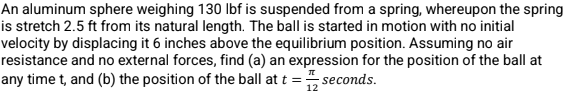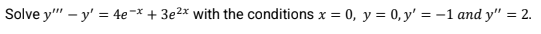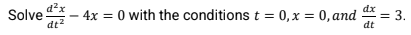# Systems of Linear Equations - Learn the Basics

Recent questions in Second order linear equationsAydin Welch 2022-12-20

## Find the general solution of the given differential equation:${y}^{″}-2{y}^{\prime }+y=0$blackdivcp 2022-10-23

## One solution of the different equation ${y}^{″}+{y}^{\prime }=0$ is $y={e}^{-x}$. Use Reduction of Order to find a second linearly independent solution.LEIDY KATERINE HERRERA AMORTEGUI2022-08-11

## dx/dy=-(4y^2+6xy/3y^2+2xcapellitad9 2022-07-17

## If $y=x{e}^{7x}$ is a solution of a linear homogeneous 2nd order DE, then another solution might be?Bacille John Purca2022-05-18

##Bacille John Purca2022-05-18

##Bacille John Purca2022-05-18

##Amya Horn 2022-03-31

## Solve the differential equation by using method of variation of parametrs$y{}^{‴}+9{y}^{\prime }=\frac{1}{f\left(x\right)}$take $f\left(x\right)=\mathrm{cos}11x$wibawanyacl3q 2022-03-29

## Solve differential equation.$y{}^{″}+2{y}^{\prime }=3{x}^{2}+2x$Kendall Daniels 2022-03-28

## A nonhomogeneous second-order linear equation and a complementary function ${y}_{c}$ are given. Find a particular solution of the equation.Reuben Brennan 2022-03-25

## Find a second-order lincar equation for which $y\left(x\right)={c}_{1}{e}^{3x}+{c}_{2}{e}^{5x}+\mathrm{sin}\left(2x\right)$is the general solutionCoradossi7xod 2022-03-25

## Consider the following linear second-order homogeneous differential equation with constant coefficients and two initial conditionsBlackettyl2j 2022-03-25

## Solve the following linear second order homogeneous differential equationPizzadililehz 2022-03-25

## Find the general solution of the given differential equations$4y{}^{″}-4{y}^{\prime }-3=0$Destinee Hensley 2022-03-25

## Find the general solution of the given second-order differential equations$2y{}^{″}+2{y}^{\prime }+y=0$kembdumatxf 2022-03-25

## Find the general solution to the following second order differential equations.$y{}^{″}-{6}^{\prime }+25y=0$avalg10o 2022-03-25

## Find a particular solution to the differential equation.$y{}^{″}-{y}^{\prime }-6y=\mathrm{sin}t+3\mathrm{cos}t$Jackson Floyd 2022-03-25

## Find ODE having the given function as its general solution.$y={x}^{2}+{c}_{1}{e}^{2x}+{c}_{2}{e}^{3x}$Asher Olsen 2022-03-24
## Solve differential equation.$y{}^{″}-15{y}^{\prime }+56y=0$ideklaraz7xz 2022-03-24